# ISEE Upper Level Math : How to find an angle in a rhombus

## Example Questions

### Example Question #1 : How To Find An Angle In A Rhombus

Consider the rhombus below. Solve for.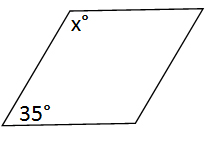Possible Answers: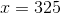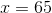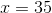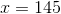Correct answer:Explanation:

The total sum of the interior angles of a quadrilateral is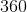degrees. In this problem, we are only considering half of the interior angles: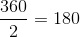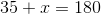### Example Question #2 : How To Find An Angle In A Rhombus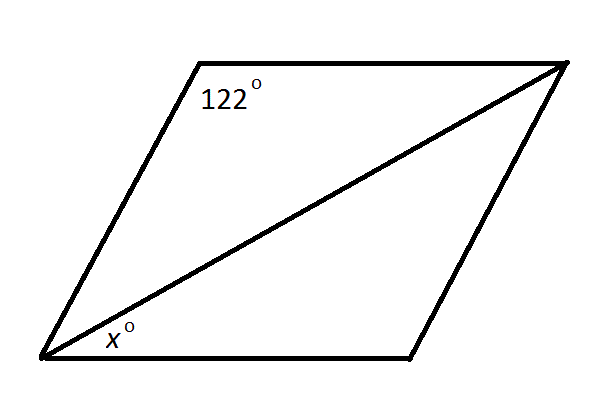Note: Figure NOT drawn to scale.

The above depicts a rhombus and one of its diagonals. What is?

Possible Answers: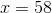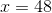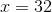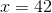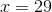Correct answer:Explanation:

The diagonals of a rhombus bisect the angles.

The angle bisected must be supplementary to the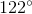angle since they are consecutive angles of a parallelogram; therefore, that angle has measure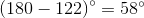, andis half that, or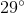.

### All ISEE Upper Level Math Resources